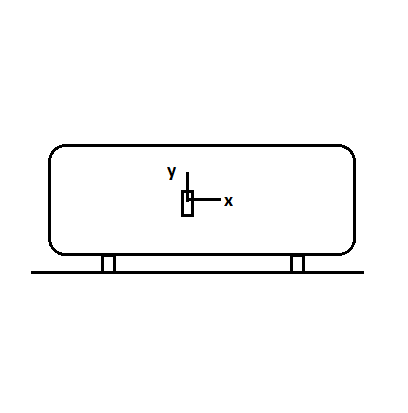# A strain gage is mounted to the outer surface of a thin-walled boiler as shown. The boiler has an...

## Question:

A strain gage is mounted to the outer surface of a thin-walled boiler as shown. The boiler has an inside diameter of 37.5 in. and a wall thickness of 1.000 in., and it is made of stainless steel E = 28,000 ksi;? = 0.27. Determine the internal pressure in the boiler when the strain gage reads 100 ?? in the y-direction.## Stresses in Pressure Vessels:

Hoop Stress:

The hoop stress also called the circumferential stress induced in the boilers or pressure vessels can be found from the relation,

{eq}\sigma_c=\dfrac {pd}{2t} {/eq}

Longitudinal Stress:

The longitudinal stress induced in the boilers or pressure vessels can be found from the relation,

{eq}\sigma_l=\dfrac {pd}{4t} {/eq}

Where, 'p', 'd' and 't' are internal pressure, diameter and the thickness of the pressure vessel respectively.

Poisson's Ratio:

The ratio of the lateral strain to the axial strain is known as Poisson's ratio.

For most of the material, the value of Poisson's ratio varies from 0.25 to 0.33.

Find the ratio of {eq}\dfrac {d}{t}=\dfrac {37.5}{1}=37.5 {/eq}.

Since the ratio of the diameter to the thickness is greater than 20, the vessel...

Become a Study.com member to unlock this answer! Create your account